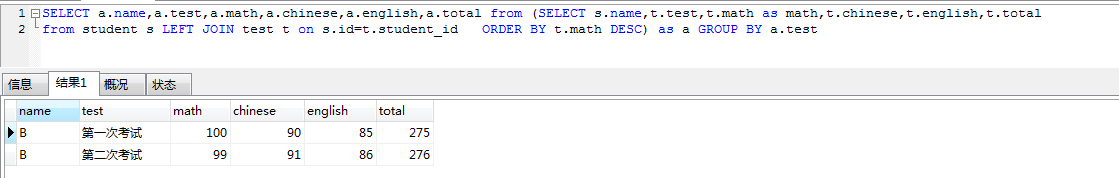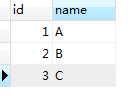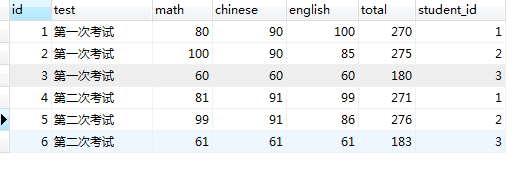sql語句：SELECT s.name,t.test,t.math,t.chinese,t.english,max(t.total) from student s LEFT JOIN test t on s.id=t.student_id GROUP BY t.test

sql語句：SELECT s.name,t.test,max(t.math),t.chinese,t.english,t.total from student s LEFT JOIN test t on s.id=t.student_id GROUP BY t.test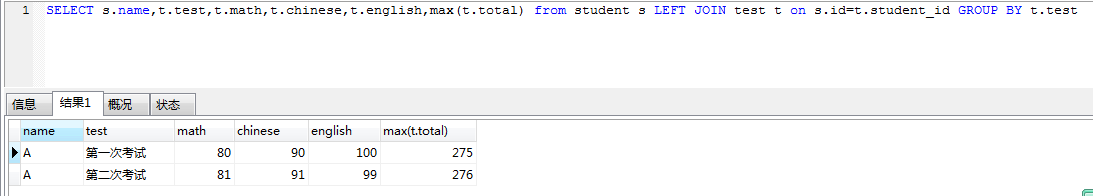group by預設返回每一組的第一條資料（每一組的資料排序都是按預設順序排序的），所以第一次查詢結果正確完全是巧合！（SQL錯了哈）

SQL語句：SELECT s.name,t.test,t.math,t.chinese,t.english,t.total from student s LEFT JOIN test t on s.id=t.student_id ORDER BY t.math DESC GROUP BY t.test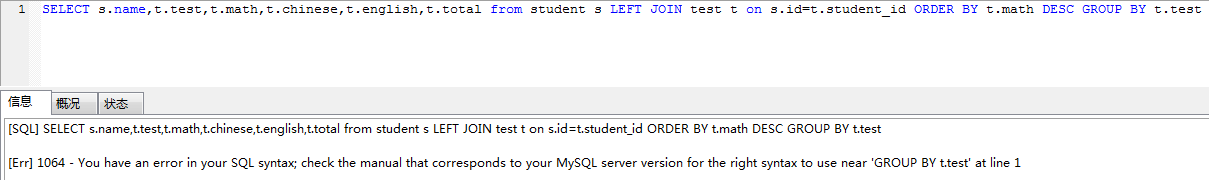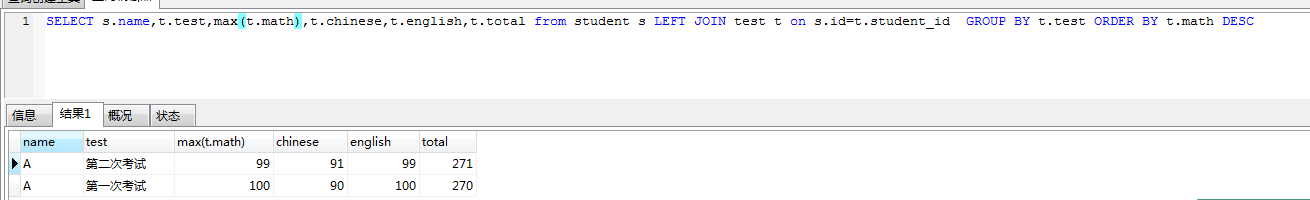SQL語句：SELECT a.name,a.test,a.math,a.chinese,a.english,a.total from (SELECT s.name,t.test,t.math as math,t.chinese,
t.english,t.total   from student s LEFT JOIN test t on s.id=t.student_id   ORDER BY t.math DESC) as a GROUP BY a.test